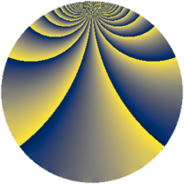# Properties

 Label 3332.1.oLevel $3332$ Weight $1$ Character orbit 3332.o Rep. character $\chi_{3332}(67,\cdot)$ Character field $\Q(\zeta_{6})$ Dimension $32$ Newform subspaces $7$ Sturm bound $504$ Trace bound $13$

# Related objects

## Defining parameters

 Level: $$N$$ $$=$$ $$3332 = 2^{2} \cdot 7^{2} \cdot 17$$ Weight: $$k$$ $$=$$ $$1$$ Character orbit: $$[\chi]$$ $$=$$ 3332.o (of order $$6$$ and degree $$2$$) Character conductor: $$\operatorname{cond}(\chi)$$ $$=$$ $$476$$ Character field: $$\Q(\zeta_{6})$$ Newform subspaces: $$7$$ Sturm bound: $$504$$ Trace bound: $$13$$

## Dimensions

The following table gives the dimensions of various subspaces of $$M_{1}(3332, [\chi])$$.

Total New Old
Modular forms 80 48 32
Cusp forms 48 32 16
Eisenstein series 32 16 16

The following table gives the dimensions of subspaces with specified projective image type.

$$D_n$$ $$A_4$$ $$S_4$$ $$A_5$$
Dimension 32 0 0 0

## Trace form

 $$32 q + 2 q^{2} - 6 q^{4} - 4 q^{8} - 12 q^{9} + O(q^{10})$$ $$32 q + 2 q^{2} - 6 q^{4} - 4 q^{8} - 12 q^{9} - 6 q^{16} + 4 q^{17} + 2 q^{18} + 4 q^{25} - 4 q^{26} + 10 q^{30} - 8 q^{32} - 4 q^{33} - 16 q^{36} - 24 q^{50} + 10 q^{60} + 12 q^{64} + 8 q^{66} + 4 q^{68} + 8 q^{69} + 12 q^{72} - 12 q^{81} - 10 q^{86} + 4 q^{93} + O(q^{100})$$

## Decomposition of $$S_{1}^{\mathrm{new}}(3332, [\chi])$$ into newform subspaces

Label Dim $A$ Field Image CM RM Traces $q$-expansion
$a_{2}$ $a_{3}$ $a_{5}$ $a_{7}$
3332.1.o.a $2$ $1.663$ $$\Q(\sqrt{-3})$$ $D_{3}$ $$\Q(\sqrt{-17})$$ None $$-1$$ $$-1$$ $$0$$ $$0$$ $$q+\zeta_{6}^{2}q^{2}-\zeta_{6}q^{3}-\zeta_{6}q^{4}+q^{6}+q^{8}+\cdots$$
3332.1.o.b $2$ $1.663$ $$\Q(\sqrt{-3})$$ $D_{3}$ $$\Q(\sqrt{-17})$$ None $$-1$$ $$1$$ $$0$$ $$0$$ $$q+\zeta_{6}^{2}q^{2}+\zeta_{6}q^{3}-\zeta_{6}q^{4}-q^{6}+q^{8}+\cdots$$
3332.1.o.c $2$ $1.663$ $$\Q(\sqrt{-3})$$ $D_{2}$ $$\Q(\sqrt{-1})$$, $$\Q(\sqrt{-17})$$ $$\Q(\sqrt{17})$$ $$1$$ $$0$$ $$0$$ $$0$$ $$q-\zeta_{6}^{2}q^{2}-\zeta_{6}q^{4}-q^{8}-\zeta_{6}^{2}q^{9}+\cdots$$
3332.1.o.d $2$ $1.663$ $$\Q(\sqrt{-3})$$ $D_{2}$ $$\Q(\sqrt{-1})$$, $$\Q(\sqrt{-17})$$ $$\Q(\sqrt{17})$$ $$1$$ $$0$$ $$0$$ $$0$$ $$q-\zeta_{6}^{2}q^{2}-\zeta_{6}q^{4}-q^{8}-\zeta_{6}^{2}q^{9}+\cdots$$
3332.1.o.e $4$ $1.663$ $$\Q(\zeta_{12})$$ $D_{2}$ $$\Q(\sqrt{-1})$$, $$\Q(\sqrt{-119})$$ $$\Q(\sqrt{119})$$ $$-2$$ $$0$$ $$0$$ $$0$$ $$q-\zeta_{12}^{2}q^{2}+\zeta_{12}^{4}q^{4}+\zeta_{12}^{5}q^{5}+\cdots$$
3332.1.o.f $4$ $1.663$ $$\Q(\zeta_{12})$$ $D_{6}$ $$\Q(\sqrt{-17})$$ None $$2$$ $$0$$ $$0$$ $$0$$ $$q+\zeta_{12}^{2}q^{2}+(-\zeta_{12}^{3}-\zeta_{12}^{5})q^{3}+\cdots$$
3332.1.o.g $16$ $1.663$ $$\Q(\zeta_{60})$$ $D_{10}$ $$\Q(\sqrt{-119})$$ None $$2$$ $$0$$ $$0$$ $$0$$ $$q+\zeta_{60}^{4}q^{2}+(-\zeta_{60}^{11}-\zeta_{60}^{29})q^{3}+\cdots$$

## Decomposition of $$S_{1}^{\mathrm{old}}(3332, [\chi])$$ into lower level spaces

$$S_{1}^{\mathrm{old}}(3332, [\chi]) \cong$$ $$S_{1}^{\mathrm{new}}(476, [\chi])$$$$^{\oplus 2}$$